Courses

# Relational Algebra Notes | EduRev

## GATE : Relational Algebra Notes | EduRev

The document Relational Algebra Notes | EduRev is a part of the GATE Course Database Management System (DBMS).
All you need of GATE at this link: GATE

Introduction

Relational Algebra is procedural query language, which takes Relation as input and generate relation as output. Relational algebra mainly provides theoretical foundation for relational databases and SQL.

Operators in Relational Algebra
1. Projection (π): Projection is used to project required column data from a relation.
Example: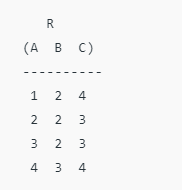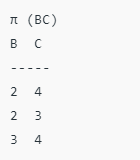Note: By Default projection removes duplicate data.
2. Selection (σ): Selection is used to select required tuples of the relations.
for the above relation
σ (c > 3)R
will select the tuples which have c more than 3.
Note: selection operator only selects the required tuples but does not display them. For displaying, data projection operator is used.
For the above selected tuples, to display we need to use projection also.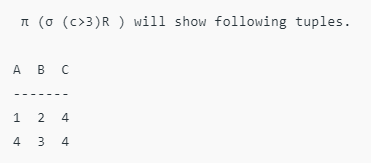3. Union (U): Union operation in relational algebra is same as union operation in set theory, only constraint is for union of two relation both relation must have same set of Attributes.
4. Set Difference (-): Set Difference in relational algebra is same set difference operation as in set theory with the constraint that both relation should have same set of attributes.
5. Rename (ρ): Rename is a unary operation used for renaming attributes of a relation.
ρ (a/b)R will rename the attribute ‘b’ of relation by ‘a’.
6. Cross Product (X)
Cross product between two relations let say A and B, so cross product between A X B will results all the attributes of A followed by each attribute of B. Each record of A will pairs with every record of B.
below is the example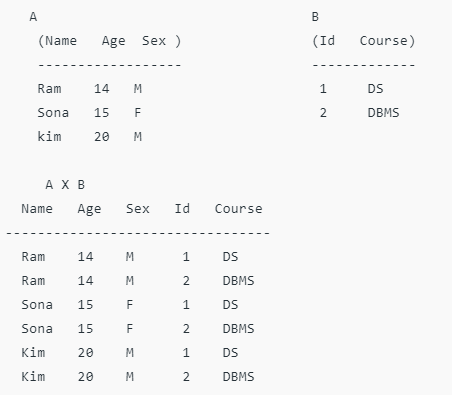Note: if A has ‘n’ tuples and B has ‘m’ tuples then A X B will have ‘n*m’ tuples.
7. Natural Join (⋈): Natural join is a binary operator. Natural join between two or more relations will result set of all combination of tuples where they have equal common attribute.
Let us see below example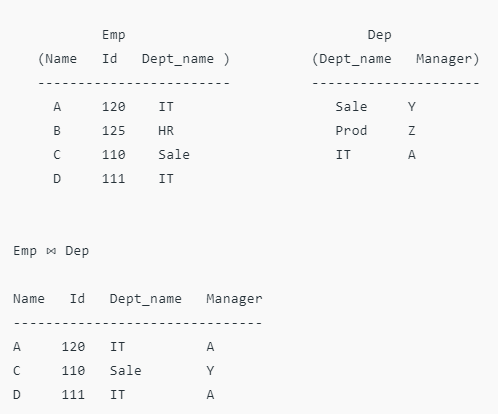8. Conditional Join: Conditional join works similar to natural join. In natural join, by default condition is equal between common attribute while in conditional join we can specify the any condition such as greater than, less than, not equal
Let us see below example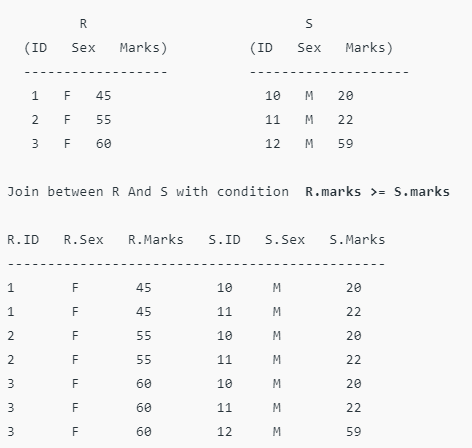Offer running on EduRev: Apply code STAYHOME200 to get INR 200 off on our premium plan EduRev Infinity!

49 docs|15 tests

,

,

,

,

,

,

,

,

,

,

,

,

,

,

,

,

,

,

,

,

,

;